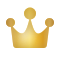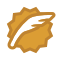ADCensusAD-Census

## 代码实现

### 类设计

#### 成员函数

/**
* \brief 十字交叉域代价聚合器
*/
class CrossAggregator {
public:
CrossAggregator();
~CrossAggregator();
}


/**
* \brief 初始化代价聚合器
* \param width		影像宽
* \param height	影像高
* \return true:初始化成功
*/
bool Initialize(const sint32& width, const sint32& height, const sint32& min_disparity, const sint32& max_disparity);

/**
* \brief 设置代价聚合器的数据
* \param img_left		// 左影像数据，三通道
* \param img_right		// 右影像数据，三通道
* \param cost_init		// 初始代价数组
*/
void SetData(const uint8* img_left, const uint8* img_right, const float32* cost_init);

/**
* \brief 设置代价聚合器的参数
* \param cross_L1		// L1
* \param cross_L2		// L2
* \param cross_t1		// t1
* \param cross_t2		// t2
*/
void SetParams(const sint32& cross_L1, const sint32& cross_L2, const sint32& cross_t1, const sint32& cross_t2);

/** \brief 聚合 */
void Aggregate(const sint32& num_iters);

/** \brief 获取所有像素的十字交叉臂数据指针 */
CrossArm* get_arms_ptr();

/** \brief 获取聚合代价数组指针 */
float32* get_cost_ptr();


/**
* \brief 交叉十字臂结构
* 为了限制内存占用，臂长类型设置为uint8，这意味着臂长最长不能超过255
*/
struct CrossArm {
uint8 left, right, top, bottom;
CrossArm() : left(0), right(0), top(0), bottom(0) { }
};


1. 构建十字交叉臂
2. 计算像素的支持区像素数量
3. 聚合所有像素在某固定视差下的代价

/** \brief 构建十字交叉臂 */
void BuildArms();
/** \brief 搜索水平臂 */
void FindHorizontalArm(const sint32& x, const sint32& y, uint8& left, uint8& right) const;
/** \brief 搜索竖直臂 */
void FindVerticalArm(const sint32& x, const sint32& y, uint8& top, uint8& bottom) const;
/** \brief 计算像素的支持区像素数量 */
void ComputeSupPixelCount();
/** \brief 聚合某个视差 */
void AggregateInArms(const sint32& disparity, const bool& horizontal_first);


#### 成员变量

/** \brief 图像尺寸 */
sint32	width_;
sint32	height_;

/** \brief 交叉臂 */
vector<CrossArm> vec_cross_arms_;

/** \brief 影像数据 */
const uint8* img_left_;
const uint8* img_right_;

/** \brief 初始代价数组指针 */
const float32* cost_init_;
/** \brief 聚合代价数组 */
vector<float32> cost_aggr_;

/** \brief 临时代价数据 */
vector<float32> vec_cost_tmp_;
/** \brief 支持区像素数量数组 0：水平臂优先 1：竖直臂优先 */
vector<uint16> vec_sup_count_;
vector<uint16> vec_sup_count_tmp_;

sint32	cross_L1_;			// 十字交叉窗口的空间域参数：L1
sint32  cross_L2_;			// 十字交叉窗口的空间域参数：L2
sint32	cross_t1_;			// 十字交叉窗口的颜色域参数：t1
sint32  cross_t2_;			// 十字交叉窗口的颜色域参数：t2
sint32  min_disparity_;			// 最小视差
sint32	max_disparity_;			// 最大视差

/** \brief 是否成功初始化标志	*/
bool is_initialized_;


### 类实现

bool CrossAggregator::Initialize(const sint32& width, const sint32& height, const sint32& min_disparity, const sint32& max_disparity)
{
width_ = width;
height_ = height;
min_disparity_ = min_disparity;
max_disparity_ = max_disparity;

const sint32 img_size = width_ * height_;
const sint32 disp_range = max_disparity_ - min_disparity_;
if (img_size <= 0 || disp_range <= 0) {
is_initialized_ = false;
return is_initialized_;
}

// 为交叉十字臂数组分配内存
vec_cross_arms_.clear();
vec_cross_arms_.resize(img_size);

// 为临时代价数组分配内存
vec_cost_tmp_.clear();
vec_cost_tmp_.resize(img_size);
vec_cost_tmp_.clear();
vec_cost_tmp_.resize(img_size);

// 为存储每个像素支持区像素数量的数组分配内存
vec_sup_count_.clear();
vec_sup_count_.resize(img_size);
vec_sup_count_.clear();
vec_sup_count_.resize(img_size);
vec_sup_count_tmp_.clear();
vec_sup_count_tmp_.resize(img_size);

// 为聚合代价数组分配内存
cost_aggr_.resize(img_size * disp_range);

is_initialized_ = !vec_cross_arms_.empty() && !vec_cost_tmp_.empty() && !vec_cost_tmp_.empty()
&& !vec_sup_count_.empty() && !vec_sup_count_.empty()
&& !vec_sup_count_tmp_.empty() && !cost_aggr_.empty();
return is_initialized_;
}


1. 构建聚合区域，也就是构建十字交叉臂
2. 执行聚合

void CrossAggregator::FindHorizontalArm(const sint32& x, const sint32& y, uint8& left, uint8& right) const
{
// 像素数据地址
const auto img0 = img_left_ + y * width_ * 3 + 3 * x;
// 像素颜色值

left = right = 0;
//计算左右臂,先左臂后右臂
sint32 dir = -1;
for (sint32 k = 0; k < 2; k++) {
// 延伸臂直到条件不满足
// 臂长不得超过cross_L1
auto img = img0 + dir * 3;
auto color_last = color0;
sint32 xn = x + dir;
for (sint32 n = 0; n < std::min(cross_L1_, MAX_ARM_LENGTH); n++) {

// 边界处理
if (k == 0) {
if (xn < 0) {
break;
}
}
else {
if (xn == width_) {
break;
}
}

// 获取颜色值

// 颜色距离1（臂上像素和计算像素的颜色距离）
const sint32 color_dist1 = ColorDist(color, color0);
if (color_dist1 >= cross_t1_) {
break;
}

// 颜色距离2（臂上像素和前一个像素的颜色距离）
if (n > 0) {
const sint32 color_dist2 = ColorDist(color, color_last);
if (color_dist2 >= cross_t1_) {
break;
}
}

// 臂长大于L2后，颜色距离阈值减小为t2
if (n + 1 > cross_L2_) {
if (color_dist1 >= cross_t2_) {
break;
}
}

if (k == 0) {
left++;
}
else {
right++;
}
color_last = color;
xn += dir;
img += dir * 3;
}
dir = -dir;
}
}


void CrossAggregator::ComputeSupPixelCount()
{
// 计算每个像素的支持区像素数量
// 注意：两种不同的聚合方向，像素的支持区像素是不同的，需要分开计算
bool horizontal_first = true;
for (sint32 n = 0; n < 2; n++) {
// n=0 : horizontal_first; n=1 : vertical_first
const sint32 id = horizontal_first ? 0 : 1;
for (sint32 k = 0; k < 2; k++) {
// k=0 : pass1; k=1 : pass2
for (sint32 y = 0; y < height_; y++) {
for (sint32 x = 0; x < width_; x++) {
// 获取arm数值
auto& arm = vec_cross_arms_[y*width_ + x];
sint32 count = 0;
if (horizontal_first) {
if (k == 0) {
// horizontal
for (sint32 t = -arm.left; t <= arm.right; t++) {
count++;
}
}
else {
// vertical
for (sint32 t = -arm.top; t <= arm.bottom; t++) {
count += vec_sup_count_tmp_[(y + t)*width_ + x];
}
}
}
else {
if (k == 0) {
// vertical
for (sint32 t = -arm.top; t <= arm.bottom; t++) {
count++;
}
}
else {
// horizontal
for (sint32 t = -arm.left; t <= arm.right; t++) {
count += vec_sup_count_tmp_[y*width_ + x + t];
}
}
}
if (k == 0) {
vec_sup_count_tmp_[y*width_ + x] = count;
}
else {
vec_sup_count_[id][y*width_ + x] = count;
}
}
}
}
horizontal_first = !horizontal_first;
}
}


void CrossAggregator::AggregateInArms(const sint32& disparity, const bool& horizontal_first)
{
// 此函数聚合所有像素当视差为disparity时的代价

if (disparity < min_disparity_ || disparity >= max_disparity_) {
return;
}
const auto disp = disparity - min_disparity_;
const sint32 disp_range = max_disparity_ - min_disparity_;
if (disp_range <= 0) {
return;
}

// 将disp层的代价存入临时数组vec_cost_tmp_
// 这样可以避免过多的访问更大的cost_aggr_,提高访问效率
for (sint32 y = 0; y < height_; y++) {
for (sint32 x = 0; x < width_; x++) {
vec_cost_tmp_[y * width_ + x] = cost_aggr_[y * width_ * disp_range + x * disp_range + disp];
}
}

// 逐像素聚合
const sint32 ct_id = horizontal_first ? 0 : 1;
for (sint32 k = 0; k < 2; k++) {
// k==0: pass1
// k==1: pass2
for (sint32 y = 0; y < height_; y++) {
for (sint32 x = 0; x < width_; x++) {
// 获取arm数值
auto& arm = vec_cross_arms_[y*width_ + x];
// 聚合
float32 cost = 0.0f;
if (horizontal_first) {
if (k == 0) {
// horizontal
for (sint32 t = -arm.left; t <= arm.right; t++) {
cost += vec_cost_tmp_[y * width_ + x + t];
}
} else {
// vertical
for (sint32 t = -arm.top; t <= arm.bottom; t++) {
cost += vec_cost_tmp_[(y + t)*width_ + x];
}
}
}
else {
if (k == 0) {
// vertical
for (sint32 t = -arm.top; t <= arm.bottom; t++) {
cost += vec_cost_tmp_[(y + t) * width_ + x];
}
} else {
// horizontal
for (sint32 t = -arm.left; t <= arm.right; t++) {
cost += vec_cost_tmp_[y*width_ + x + t];
}
}
}
if (k == 0) {
vec_cost_tmp_[y*width_ + x] = cost;
}
else {
cost_aggr_[y*width_*disp_range + x*disp_range + disp] = cost / vec_sup_count_[ct_id][y*width_ + x];
}
}
}
}
}


void CrossAggregator::Aggregate(const sint32& num_iters)
{
if (!is_initialized_) {
return;
}

const sint32 disp_range = max_disparity_ - min_disparity_;

// 构建像素的十字交叉臂
BuildArms();

// 代价聚合
// horizontal_first 代表先水平方向聚合
bool horizontal_first = true;

// 计算两种聚合方向的各像素支持区像素数量
ComputeSupPixelCount();

// 先将聚合代价初始化为初始代价
memcpy(&cost_aggr_, cost_init_, width_*height_*disp_range*sizeof(float32));

// 多迭代聚合
for (sint32 k = 0; k < num_iters; k++) {
for (sint32 d = min_disparity_; d < max_disparity_; d++) {
AggregateInArms(d, horizontal_first);
}
// 下一次迭代，调换顺序
horizontal_first = !horizontal_first;
}
}


void ADCensusStereo::CostAggregation()
{
// 设置聚合器数据
aggregator_.SetData(img_left_, img_right_, cost_computer_.get_cost_ptr());
// 设置聚合器参数
aggregator_.SetParams(option_.cross_L1, option_.cross_L2, option_.cross_t1, option_.cross_t2);
// 代价聚合
aggregator_.Aggregate(4);
}


## 实验左视图右视图代价计算代价聚合

Ethan Li 李迎松（知乎：李迎松）

2019年获测绘科技进步一等奖（省部级）

GitHub： https://github.com/ethan-li-coding （欢迎follow和star）Ethan Li 李迎松CSDN认证博客专家 立体视觉 工学博士 博客专家

2019年国家测绘科技进步一等奖

https://github.com/ethan-li-coding（欢迎follow和star）

08-042483
10-08126908-022539
01-131万+
08-261591
03-06
11-026343
12-306798
07-2943